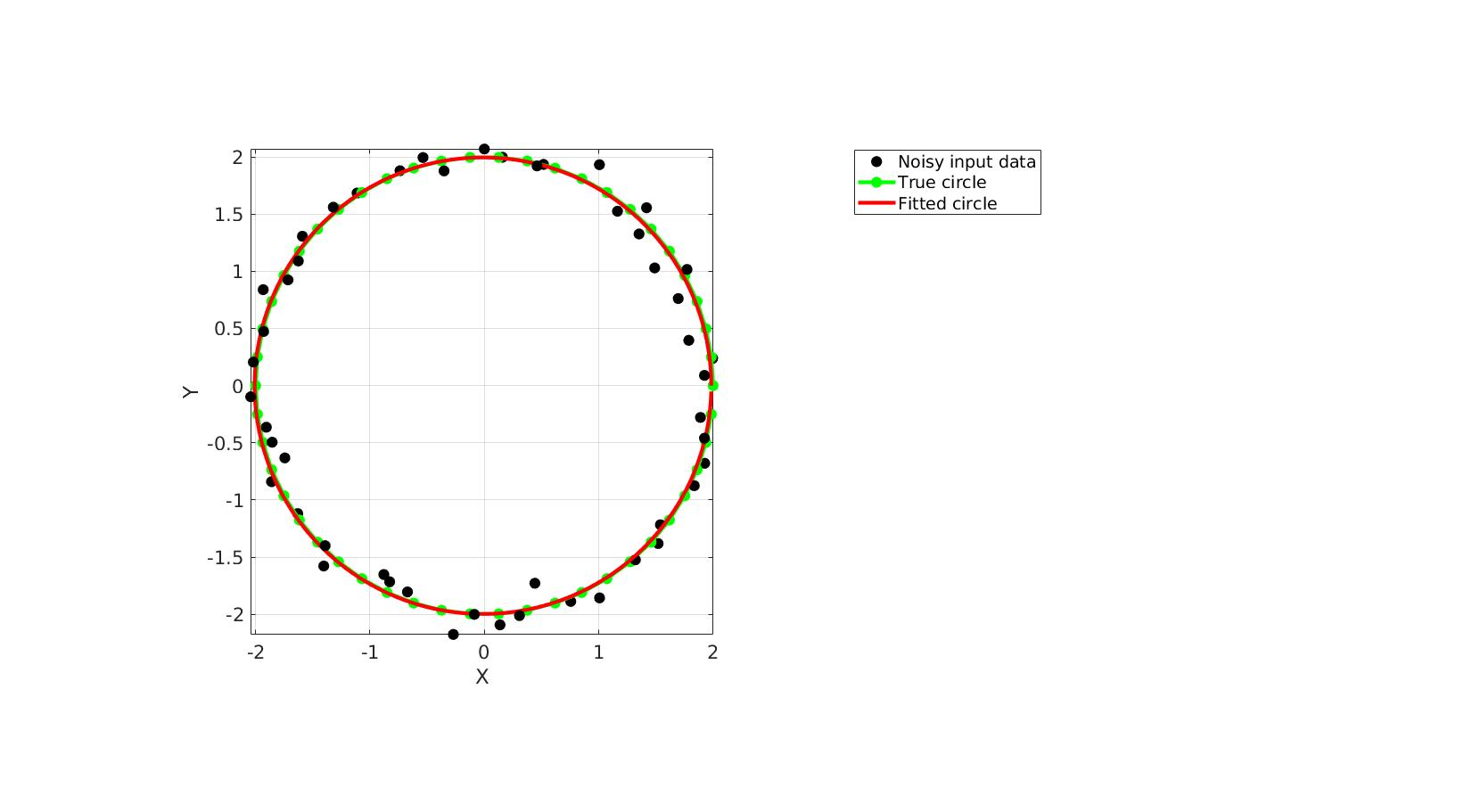# circlefit

Below is a demonstration of the features of the circlefit function

## Contents

```clear; close all; clc;
```

## Syntax

[Vc,R]=circlefit(V);

## Description

This function returns the centre Vc and radius R for a circle fitted to the input point set V. The input can be 2D or 3D but the output centre is always a point in 3D space.

## Examples

Plot settings

```markerSize=30;
lineWidth=3;
```

Create input circle

```n=50; % Number of points on the circle
t=linspace(0,2*pi,n+1)'; t=t(1:end-1); % Angles
V_true=r.*[cos(t) sin(t) zeros(size(t))]; %Circle true coordinates
V=V_true+r/20*randn(size(V_true));
```

Fit a circle

```[Vc,R]=circlefit(V);

nf=250;
r=2;
t=linspace(0,2*pi,nf+1)'; t=t(1:end-1);
Vf=Vc+R.*[cos(t) sin(t) zeros(size(t))];
```

Visualize result

```cFigure; hold on;
hp1=plotV(V,'k.','MarkerSize',markerSize);
hp2=plotV(V_true,'g.-','MarkerSize',markerSize,'LineWidth',lineWidth);
hp3=plotV(Vf,'r-','LineWidth',lineWidth);
legend([hp1 hp2 hp3],{'Noisy input data','True circle','Fitted circle'},'Location','NorthEastOutside')
axisGeom; view(2);
drawnow;
```GIBBON www.gibboncode.org

Kevin Mattheus Moerman, [email protected]

GIBBON footer text

GIBBON: The Geometry and Image-based Bioengineering add-On. A toolbox for image segmentation, image-based modeling, meshing, and finite element analysis.

Copyright (C) 2006-2022 Kevin Mattheus Moerman and the GIBBON contributors

This program is free software: you can redistribute it and/or modify it under the terms of the GNU General Public License as published by the Free Software Foundation, either version 3 of the License, or (at your option) any later version.

This program is distributed in the hope that it will be useful, but WITHOUT ANY WARRANTY; without even the implied warranty of MERCHANTABILITY or FITNESS FOR A PARTICULAR PURPOSE. See the GNU General Public License for more details.

You should have received a copy of the GNU General Public License along with this program. If not, see http://www.gnu.org/licenses/.